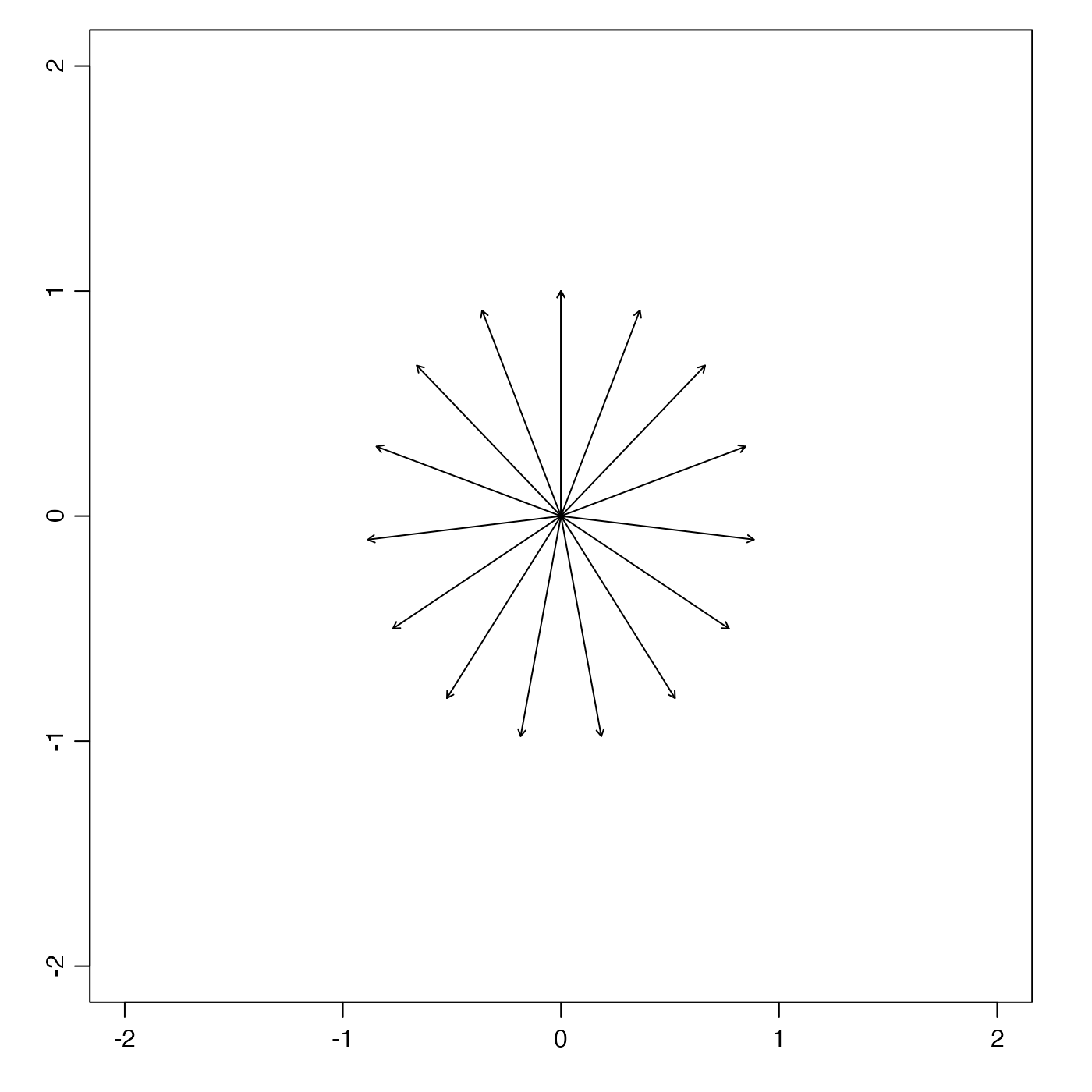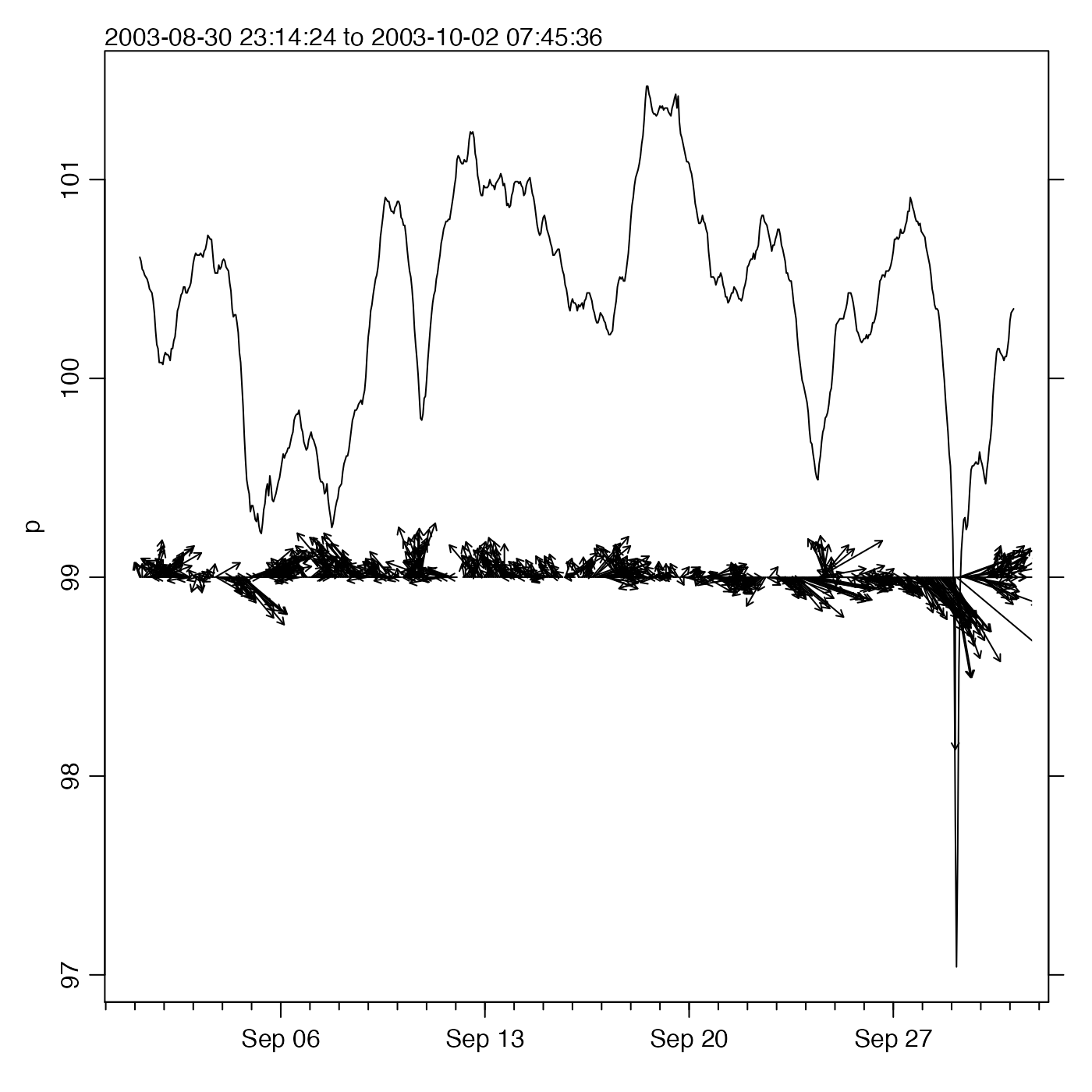The arrows are drawn with directions on the graph that match the directions indicated by the u and v components. The arrow size is set relative to the units of the y axis, according to the value of yscale, which has the unit of v divided by the unit of y. The interpretation of diagrams produced by plotSticks can be difficult, owing to overlap in the arrows. For this reason, it is often a good idea to smooth u and v before using this function.

plotSticks(
x,
y,
u,
v,
yscale = 1,
length = 1/20,
mgp = getOption("oceMgp"),
mar = c(mgp + 1, mgp + 1, 1, 1 + par("cex")),
xlab = "",
ylab = "",
col = 1,
...
)

## Arguments

x x coordinates of stick origins. y coordinates of stick origins. If not supplied, 0 will be used; if length is less than that of x, the first number is repeated and the rest are ignored. x component of stick length. y component of stick length. scale from u and v to y (see “Description”). boolean, set TRUE to add to an existing plot. value to be provided to arrows(); here, we set a default that is smaller than normally used, because these plots tend to be crowded in oceanographic applications. 3-element numerical vector to use for par("mgp"). Note that the default mar is computed from the mgp value. The default is tighter than the R default, in order to use more space for the data and less for the axes. value to be used with par("mar"). labels for the plot axes. The default is not to label them. color of sticks, in either numerical or character format. This is made to have length matching that of x by a call to rep(), which can be handy in e.g. colorizing a velocity field by day. graphical parameters passed down to arrows(). It is common, for example, to use smaller arrow heads than arrows() uses; see “Examples”.

## Examples

library(oce)

# Flow from a point source
n <- 16
x <- rep(0, n)
y <- rep(0, n)
theta <- seq(0, 2*pi, length.out=n)
u <- sin(theta)
v <- cos(theta)
plotSticks(x, y, u, v, xlim=c(-2, 2), ylim=c(-2, 2))rm(n, x, y, theta, u, v)

# Oceanographic example
data(met)
t <- met[["time"]]
u <- met[["u"]]
v <- met[["v"]]
p <- met[["pressure"]]
oce.plot.ts(t, p)plotSticks(t, 99, u, v, yscale=25, add=TRUE)